# Math in Focus Grade 4 Chapter 6 Practice 5 Answer Key Renaming Improper Fractions and Mixed Numbers

Practice the problems of Math in Focus Grade 4 Workbook Answer Key Chapter 6 Practice 5 Renaming Improper Fractions and Mixed Numbers to score better marks in the exam.

## Math in Focus Grade 4 Chapter 6 Practice 5 Answer Key Renaming Improper Fractions and Mixed Numbers

Express each improper fraction as a mixed number.

Example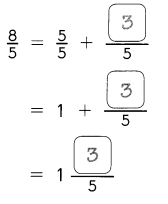Question 1.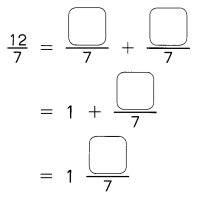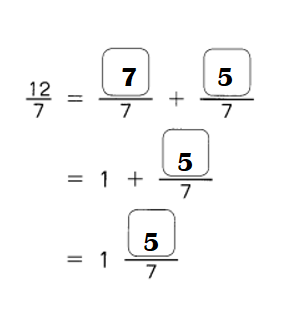Explanation:
$$\frac{12}{7}$$ = $$\frac{7}{7}$$ + $$\frac{5}{7}$$
= 1 + $$\frac{5}{7}$$
= 1$$\frac{5}{7}$$

Question 2.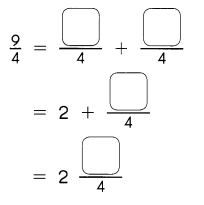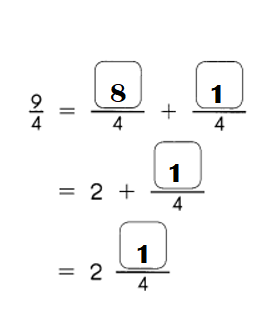Explanation:
$$\frac{9}{4}$$ = $$\frac{8}{4}$$ + $$\frac{1}{4}$$
= 2 + $$\frac{1}{4}$$
= 2$$\frac{1}{4}$$

Question 3.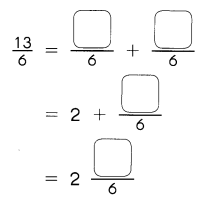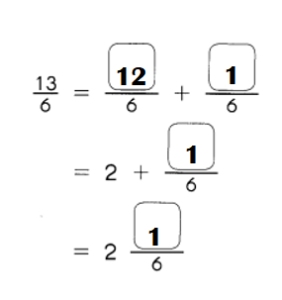Explanation:
$$\frac{13}{6}$$ = $$\frac{12}{6}$$ + $$\frac{1}{6}$$
= 2 + $$\frac{1}{6}$$
= 2$$\frac{1}{6}$$

Express each improper fraction as a whole number or a mixed number in simplest form. Show your work.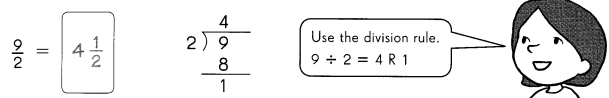Question 4.
$$\frac{17}{4}$$ = ____
$$\frac{17}{4}$$ = 4$$\frac{1}{4}$$

Explanation:
$$\frac{17}{4}$$ = 4$$\frac{1}{4}$$  {[(4 × 4) + 1] ÷ 4}

Question 5.
$$\frac{29}{6}$$ = ____
$$\frac{29}{6}$$ = 4$$\frac{5}{6}$$

Explanation:
$$\frac{29}{6}$$ = 4$$\frac{5}{6}$$ {[(4 × 6) + 5] ÷ 6}

Express each improper fraction as a whole number or a mixed number in simplest form. Show your work.

Question 6.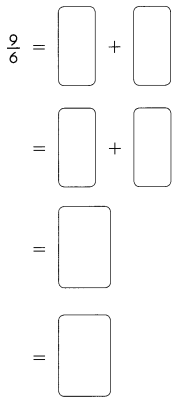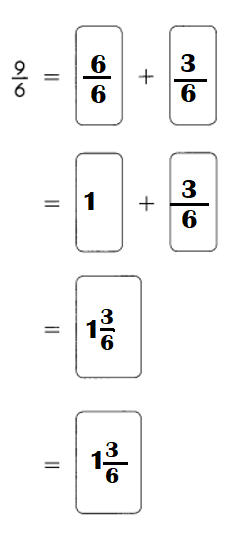Explanation:
$$\frac{9}{6}$$  =  $$\frac{6}{6}$$   + $$\frac{7}{6}$$
= 1 + $$\frac{3}{6}$$
= 1 $$\frac{3}{6}$$

Question 7.
$$\frac{12}{4}$$ = ____
$$\frac{12}{4}$$ = 3.

Explanation:
$$\frac{12}{4}$$ = $$\frac{3}{4}$$ + $$\frac{9}{4}$$
= (3 + 9) ÷ 4
= 12 ÷ 4
= 3.

Question 8.
$$\frac{21}{3}$$ = ____
$$\frac{21}{3}$$ = 7.

Explanation:
$$\frac{21}{3}$$ = $$\frac{17}{3}$$ + $$\frac{4}{3}$$
= (17 + 4) ÷ 3
= 21 ÷ 3
= 7.

Question 9.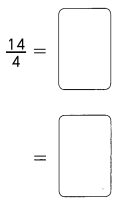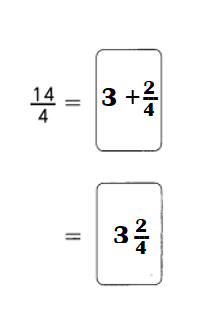Explanation:
$$\frac{14}{4}$$  = 2 + $$\frac{2}{4}$$
= 2 $$\frac{2}{4}$$

Question 10.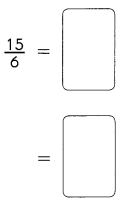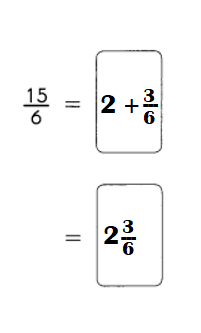Explanation:
$$\frac{15}{6}$$  = 2 + $$\frac{3}{6}$$
= 2 $$\frac{3}{6}$$

Express each mixed number as an improper fraction.

Example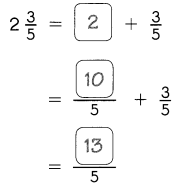Question 11.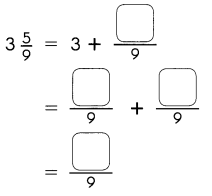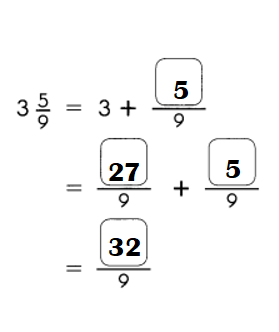Explanation:
3$$\frac{5}{9}$$ = 3 + $$\frac{5}{9}$$
=  $$\frac{27}{9}$$ + $$\frac{5}{9}$$
=  $$\frac{32}{9}$$

Question 12.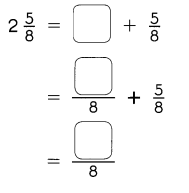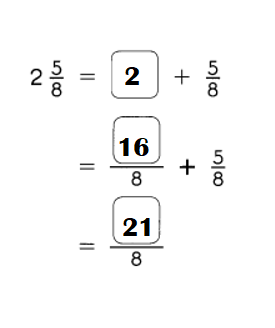Explanation:
2$$\frac{5}{8}$$ = 2 + $$\frac{5}{8}$$
=  $$\frac{16}{8}$$ + $$\frac{5}{8}$$
=  $$\frac{21}{8}$$

Question 13.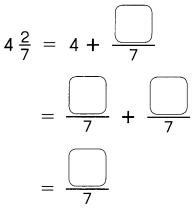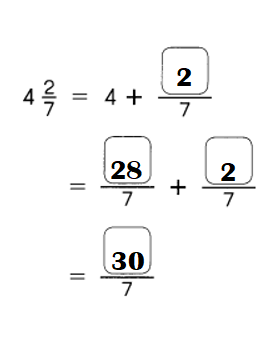Explanation:
4$$\frac{2}{7}$$ = 4 + $$\frac{2}{7}$$
=  $$\frac{28}{7}$$ + $$\frac{2}{7}$$
=  $$\frac{30}{7}$$

Express each mixed number as an improper fraction.

Example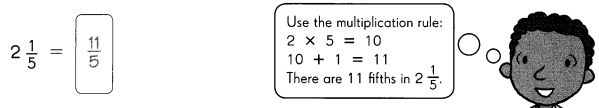Question 14.
2$$\frac{3}{8}$$ = ____
2$$\frac{3}{8}$$ = 19 ÷ 8 or $$\frac{19}{8}$$

Explanation:
2$$\frac{3}{8}$$ = (16 + 3) ÷ 8     (2 × 8 = 16)
= 19 ÷ 8 or $$\frac{19}{8}$$

Question 15.
3$$\frac{3}{4}$$ = ____
3$$\frac{3}{4}$$ = 15 ÷ 4 or $$\frac{15}{4}$$

Explanation:
3$$\frac{3}{4}$$ =  (12 + 3) ÷ 4
= 15 ÷ 4 or $$\frac{15}{4}$$

Question 16
6$$\frac{2}{5}$$ = ____
6$$\frac{2}{5}$$ = 32 ÷ 5 or $$\frac{32}{5}$$

Explanation:
6$$\frac{2}{5}$$ = (30 + 2) ÷ 5    (6 × 5 = 30)
= 32 ÷ 5 or $$\frac{32}{5}$$

Question 17.
2$$\frac{4}{7}$$ = ____
2$$\frac{4}{7}$$ = 18 ÷ 7 or $$\frac{18}{7}$$

Explanation:
2$$\frac{4}{7}$$ = (14 + 4) ÷ 7   (2 × 7 = 14)
= 18 ÷ 7 or $$\frac{18}{7}$$

Express each mixed number as an improper fraction and each improper fraction as a mixed or whole number. Then solve the riddle.

Question 18.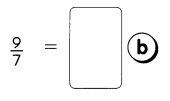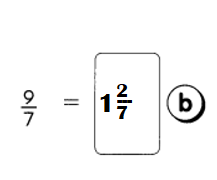Explanation:
$$\frac{9}{7}$$ = 1 + $$\frac{2}{7}$$
= 1 $$\frac{2}{7}$$

Question 19.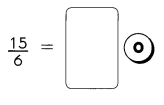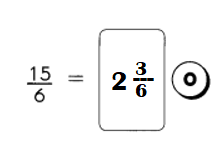Explanation:
$$\frac{15}{6}$$ = 2 + $$\frac{3}{6}$$
= 2$$\frac{3}{6}$$

Question 20.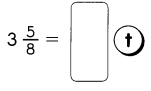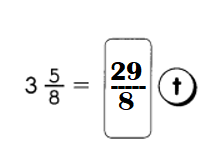Explanation:
3$$\frac{5}{8}$$ = $$\frac{24}{8}$$ + $$\frac{5}{8}$$
= (24 + 5) ÷ 8
= $$\frac{29}{8}$$ or 29 ÷ 8.

Question 21.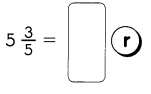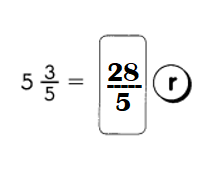Explanation:
5$$\frac{3}{5}$$ = $$\frac{25}{5}$$ + $$\frac{3}{5}$$
= (25 + 3) ÷ 5
= $$\frac{28}{5}$$ or 28 ÷ 5.

Which two animals can look behind without turning their heads? Write the letters which match the answers to find out.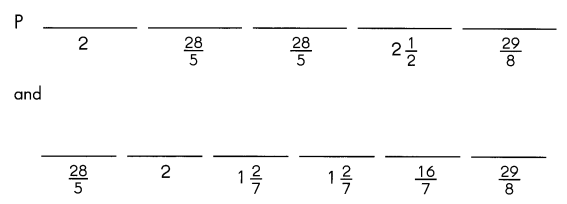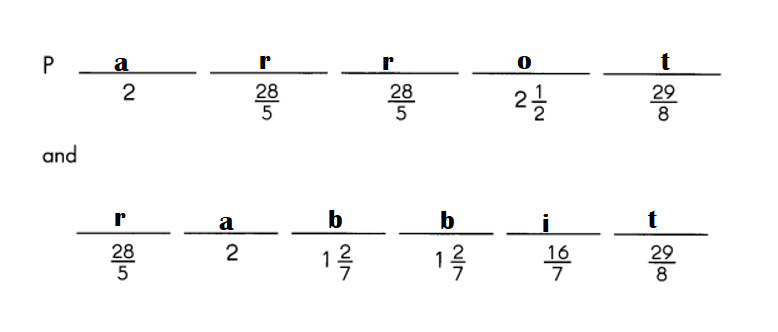$$\frac{16}{7}$$ = i.
2$$\frac{1}{2}$$ = o.